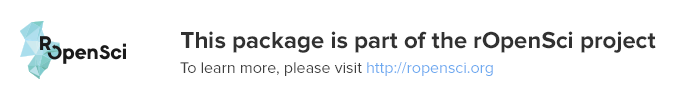# plotly v3.6.0

0

0th

Percentile

## Create Interactive Web Graphics via 'plotly.js'

Easily translate 'ggplot2' graphs to an interactive web-based version and/or create custom web-based visualizations directly from R. Once uploaded to a 'plotly' account, 'plotly' graphs (and the data behind them) can be viewed and modified in a web browser.# plotly

An R package for creating interactive web graphics via plotly.js.

## Installation

Install from CRAN:

install.packages("plotly")


Or install the latest development version (on GitHub) via devtools:

devtools::install_github("ropensci/plotly")


## Getting Started

### Web-based ggplot2 graphics

If you use ggplot2, ggplotly() converts your plots to an interactive, web-based version! It also provides sensible tooltips, which assists decoding of values encoded as visual properties in the plot.

library(plotly)
g <- ggplot(faithful, aes(x = eruptions, y = waiting)) +
stat_density_2d(aes(fill = ..level..), geom = "polygon") +
xlim(1, 6) + ylim(40, 100)
ggplotly(g)If you'd like to see how ggplotly() does in converting different ggplot2 examples, we host a plotly version of the official ggplot2 documentation. We also have some of our own examples here.

### plotly's custom R interface

plotly supports some chart types that ggplot2 doesn't (such as 3D surface, point, and line plots). You can create these (or any other plotly) charts using plot_ly().

plot_ly(z = volcano, type = "surface")For a more concise overview of the plot_ly() interface, see here. You might also find our examples and the figure reference to be helpful.

### Hooking onto plotly events

plotly.js exposes a number of 'standard' events that work consistently across plot types. It's easy to hook into these events using the event_data() function in shiny apps, as these examples demonstrate:You can also hook into these events without shiny using htmlwidgets::onRender() (example). This, however, requires JavaScript knowledge and makes it much harder, if not impossible, to coordinate views between htmlwidgets.

## Contributing

Please read through our contributing guidelines. Included are directions for opening issues, asking questions, contributing changes to plotly, and our code of conduct.## Functions in plotly

 Name Description hobbs Hobbs data plotly_build Create a 'plotly_built' object as.widget Convert a plotly object to an htmlwidget object config Set the default configuration for plotly knit_print.figure Embed a plotly figure as an iframe in a knitr doc embed_notebook Embed a plotly figure as an iframe into a IPython Notebook wind Wind data ggplotly Create plotly graphs using ggplot2 syntax get_figure Request a figure object event_data Access plotly user input event data in shiny add_trace Add a trace to a plotly visualization plotly_empty Create a complete empty plotly graph. gg2list Convert a ggplot to a list. plot_ly Initiate a plotly visualization bbox Estimate bounding box of a rotated string plotly_POST Create/Modify plotly graphs subplot View multiple plots in a single view offline Plotly Offline layout Add and/or modify layout of a plotly style Modify trace(s) to_basic Convert a geom to a "basic" geom. plotly-shiny Shiny bindings for plotly get_plot Obtain underlying data of plotly object knit_print.plotly_hash Print a plotly object in a knitr doc print.plotly_hash Print a plotly object %>% Pipe operator geom2trace Convert a "basic" geoms to a plotly.js trace. toRGB Convert R colours to RGBA hexadecimal colour values plotly Main interface to plotly last_plot Retrive and create the last plotly (or ggplot). print.plotly_built Print a 'built' plotly object print.figure Print a plotly figure object signup Create a new plotly account. knit_print.plotly_built Print a 'built' plotly object in a knitr doc mic Mic data plotly_IMAGE Create/Modify plotly images No Results!